# 用一次函数来实现瞄准线的反射

### 正文

#### 版本说明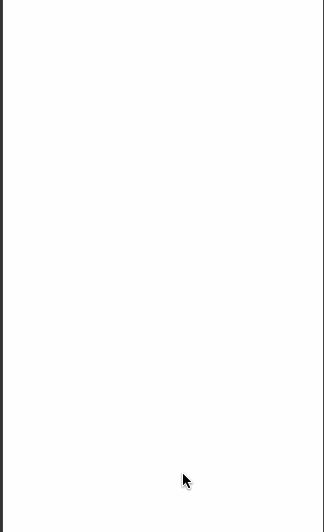#### 一次函数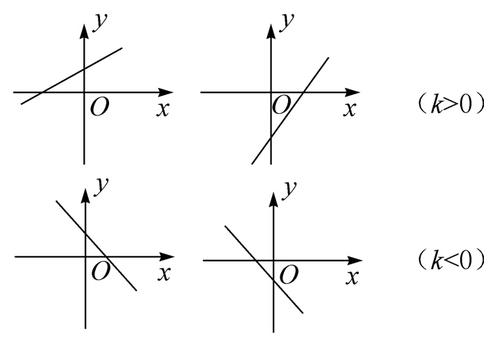#### 层级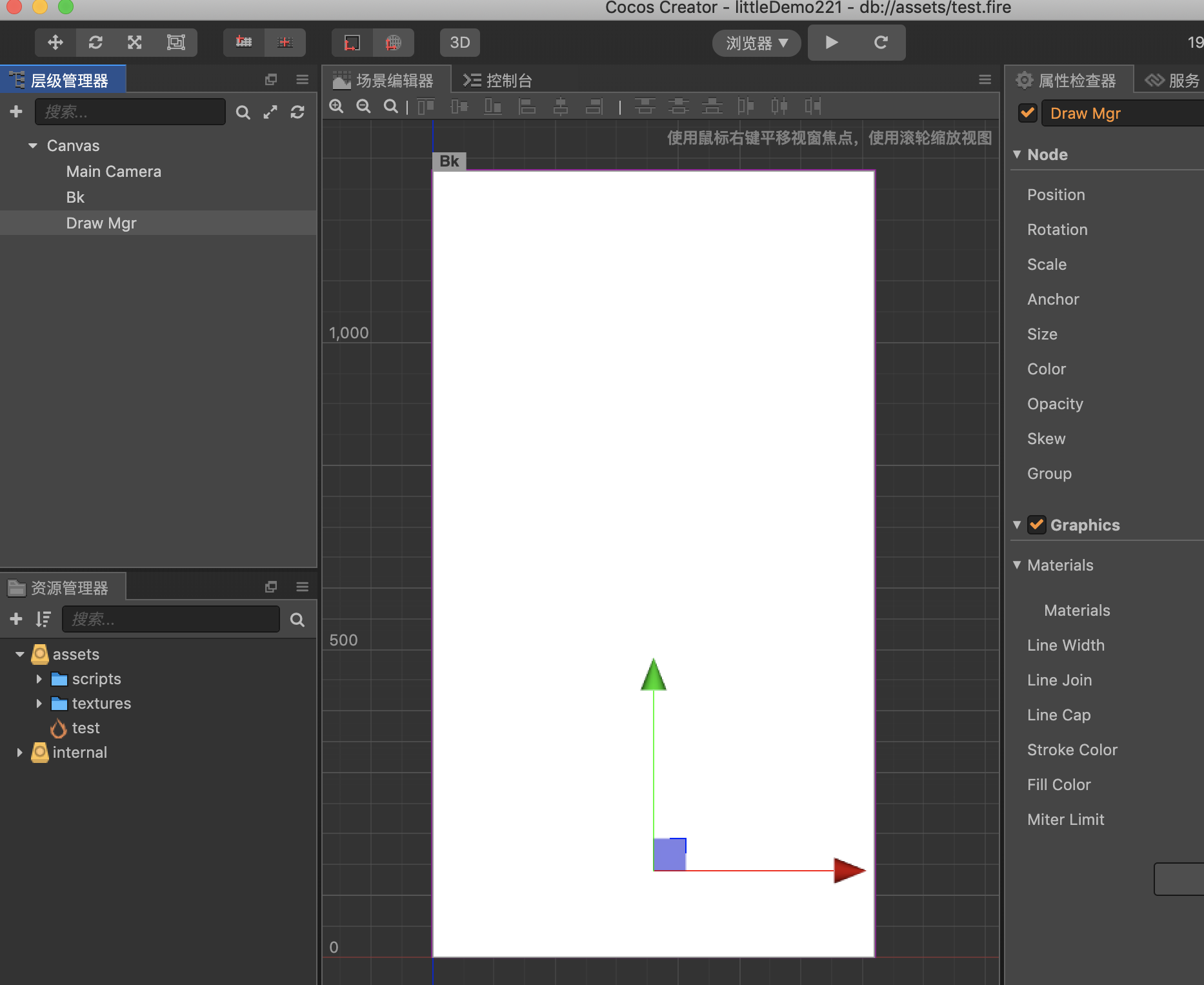#### 实现原理

/** 左右边界及总宽 */
let POS = cc.Enum({
LEFT: -360,
RIGHT: 360,
WIDTH: 720,
});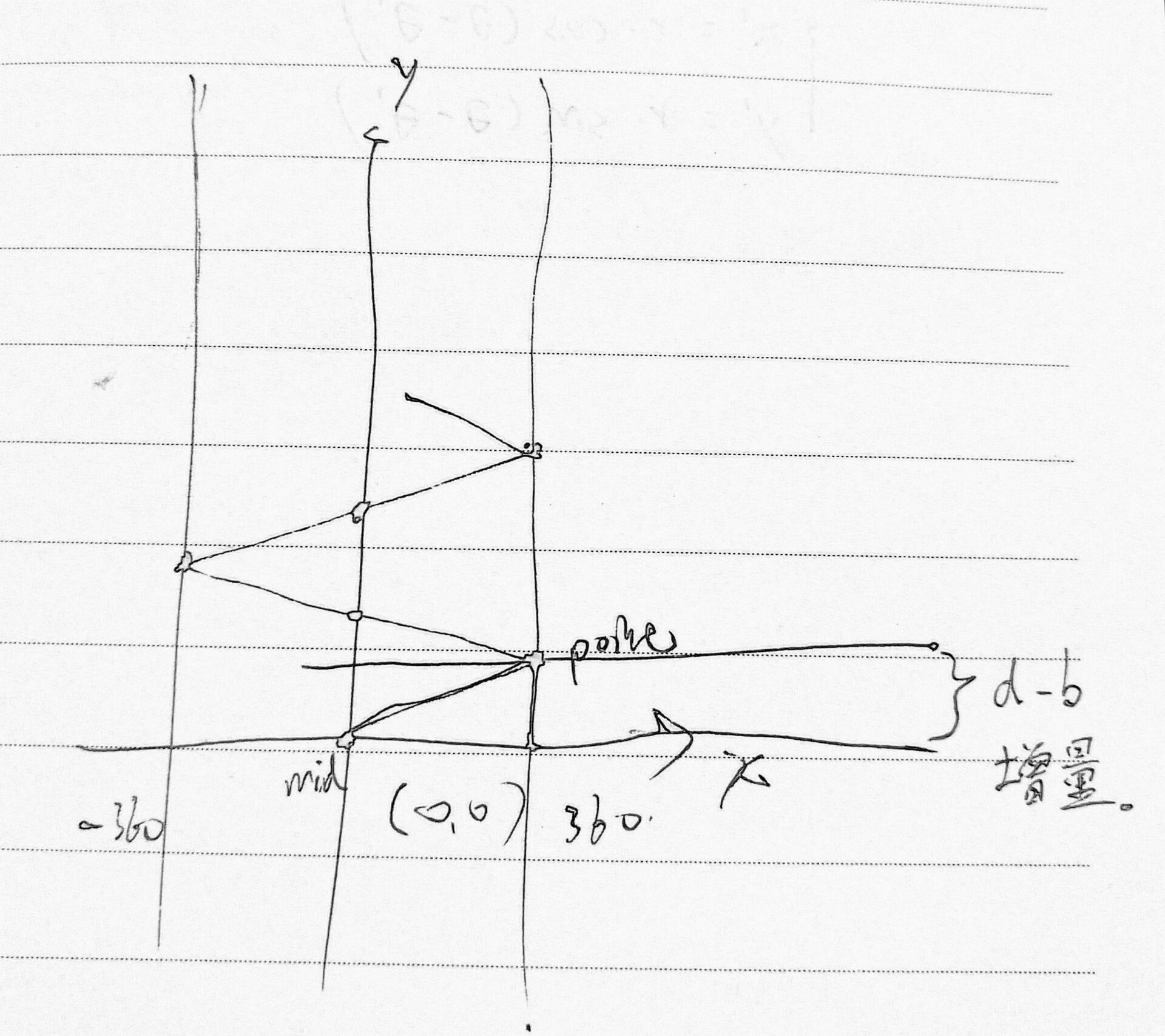// 算一下 b 的增长值
let d_b = (k > 0 ? POS.RIGHT : POS.LEFT) * k;

b = y + d_b;


#### 长度削减

drawLine (pos) {
this.draw.clear();
let lineLength = 1200;
let k = pos.y / pos.x;
// 预计到达的边界点
let point = cc.v2(0, 0);
// 画笔到起始点
this.draw.moveTo(0, 0);
let b = 0;
let x, y;
// 算一下 b 的增长值
let d_b = (k > 0 ? POS.RIGHT : POS.LEFT) * k;
// 起始标志
let isRebound = false;
while (true) {
// 如果到墙，求与墙的交点
x = k > 0 ? POS.RIGHT : POS.LEFT;
// 一元函数 y = k·x + b
y = k * x + b;
// 到达墙壁所需长度
let l = cc.v2(x, y).sub(point).mag();
// 判断能否到墙
if (l < lineLength) {
isRebound = true;
// 扣去已经过长度
lineLength -= l;
this.draw.lineTo(x, y);
// 更改下一轮循环起始点
point.x = x;
point.y = y;
b = y + d_b;
k *= -1;
} else {
// 如果不能到墙，分为两种情况，需要一个标志
if (isRebound) {
let l_k = lineLength / l;
let r_x = POS.WIDTH * l_k;
x = k > 0 ? POS.LEFT + r_x : POS.RIGHT - r_x;
y = k * x + b;
} else {
let l_k = lineLength / l;
let r_x = POS.WIDTH / 2 * l_k;
x = k > 0 ? r_x : -r_x;
y = k * x;
// 中心处理
if (x > -0.05 && x < 0.05);
y = lineLength;
}
this.draw.lineTo(x, y);
break;
}
}
this.draw.stroke();
},


#### 清除线

clearLine () {
this.draw.clear();
},


#### 触摸监听与坐标转化

start () {
this.node.on(cc.Node.EventType.TOUCH_START, (e) => {
let pos = this.node.convertToNodeSpaceAR(e.getLocation());
pos.x -= this.draw.node.x;
pos.y -= this.draw.node.y;
this.drawLine(pos);
}, this);
this.node.on(cc.Node.EventType.TOUCH_MOVE, (e) => {
let pos = this.node.convertToNodeSpaceAR(e.getLocation());
pos.x -= this.draw.node.x;
pos.y -= this.draw.node.y;
this.drawLine(pos);
}, this);
this.node.on(cc.Node.EventType.TOUCH_END, (e) => {
this.clearLine();
}, this);
},


#### 最后效果O(∩_∩)O~~

### 微信公众号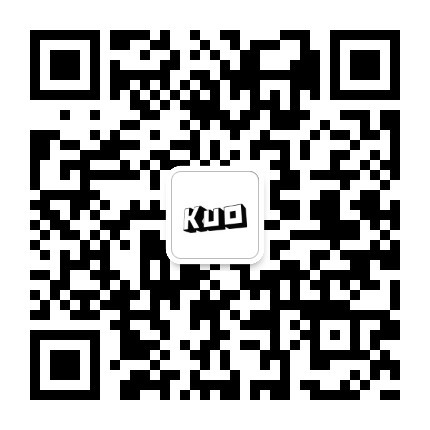©️2019 CSDN 皮肤主题: 技术工厂 设计师: CSDN官方博客